# envelope

Signal envelope

## Syntax

``[yupper,ylower] = envelope(x)``
``[yupper,ylower] = envelope(x,fl,'analytic')``
``[yupper,ylower] = envelope(x,wl,'rms')``
``[yupper,ylower] = envelope(x,np,'peak')``
``envelope(___)``

## Description

example

````[yupper,ylower] = envelope(x)` returns the upper and lower envelopes of the input sequence, `x`, as the magnitude of its analytic signal. The analytic signal of `x` is found using the discrete Fourier transform as implemented in `hilbert`. The function initially removes the mean of `x` and adds it back after computing the envelopes. If `x` is a matrix, then `envelope` operates independently over each column of `x`.```

example

````[yupper,ylower] = envelope(x,fl,'analytic')` returns the envelopes of `x` determined using the magnitude of its analytic signal. The analytic signal is computed by filtering `x` with a Hilbert FIR filter of length `fl`. This syntax is used if you specify only two arguments.```

example

````[yupper,ylower] = envelope(x,wl,'rms')` returns the upper and lower root-mean-square envelopes of `x`. The envelopes are determined using a sliding window of length `wl` samples.```

example

````[yupper,ylower] = envelope(x,np,'peak')` returns the upper and lower peak envelopes of `x`. The envelopes are determined using spline interpolation over local maxima separated by at least `np` samples.```

example

````envelope(___)` with no output arguments plots the signal and its upper and lower envelopes. This syntax accepts any of the input arguments from previous syntaxes.```

## Examples

collapse all

Generate a quadratic chirp modulated by a Gaussian. Specify a sample rate of 2 kHz and a signal duration of 2 seconds.

```t = 0:1/2000:2-1/2000; q = chirp(t-2,4,1/2,6,'quadratic',100,'convex').*exp(-4*(t-1).^2); plot(t,q)```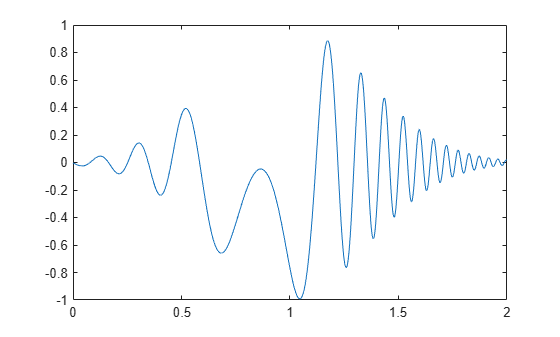Compute the upper and lower envelopes of the chirp using the analytic signal.

```[up,lo] = envelope(q); hold on plot(t,up,t,lo,'linewidth',1.5) legend('q','up','lo') hold off```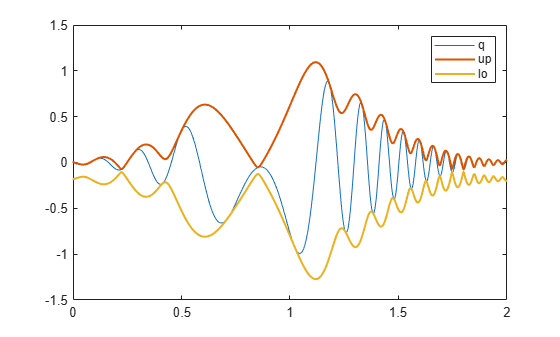The signal is asymmetric due to the nonzero mean.

Use `envelope` without output arguments to plot the signal and envelopes as a function of sample number.

`envelope(q)`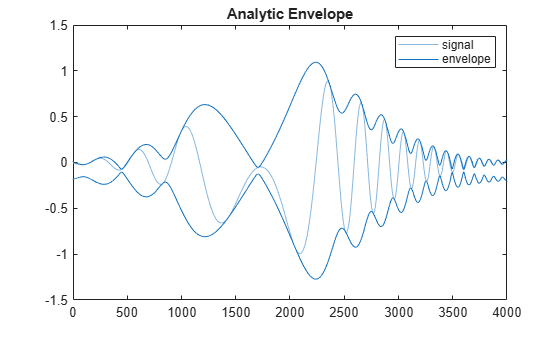Create a two-channel signal sampled at 1 kHz for 3 seconds:

• One channel is an exponentially decaying sinusoid. Specify a frequency of 7 Hz and a time constant of 2 seconds.

• The other channel is a time-displaced Gaussian-modulated chirp with a DC value of 2. Specify an initial chirp frequency of 30 Hz that decays to 5 Hz after 2 seconds.

Plot the signal.

```t = 0:1/1000:3; q1 = sin(2*pi*7*t).*exp(-t/2); q2 = chirp(t,30,2,5).*exp(-(2*t-3).^2)+2; q = [q1;q2]'; plot(t,q)```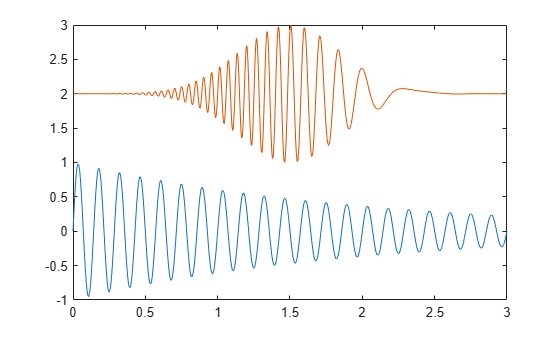Compute the upper and lower envelopes of the signal. Use a Hilbert filter with a length of 100. Plot the channels and the envelopes. Use solid lines for the upper envelopes and dashed lines for the lower envelopes.

```[up,lo] = envelope(q,100,'analytic'); hold on plot(t,up,'-',t,lo,'--') hold off```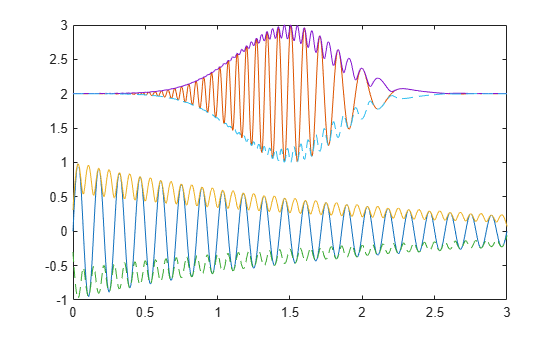Call `envelope` without output arguments to produce a plot of the signal and its envelopes as a function of sample number. Increase the filter length to 300 to obtain a smoother shape. The `'analytic'` flag is the default when you specify two input arguments.

`envelope(q,300)`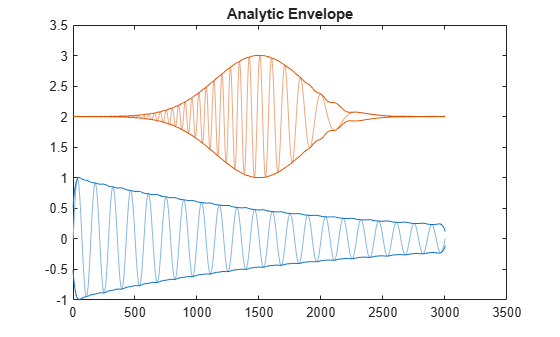Compute and plot the moving RMS envelopes of a recording of a train whistle. Use a window with a length of 150 samples.

```load('train') envelope(y,150,'rms')```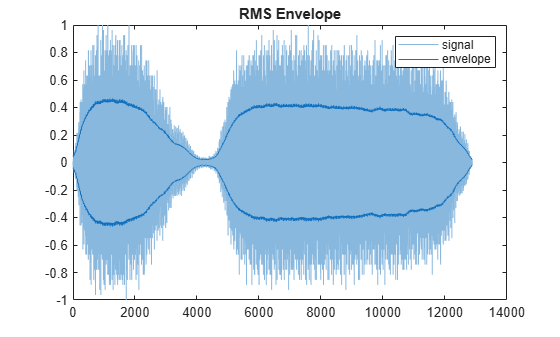Plot the upper and lower peak envelopes of a speech signal smoothed over 30-sample intervals.

```load('mtlb') envelope(mtlb,30,'peak')```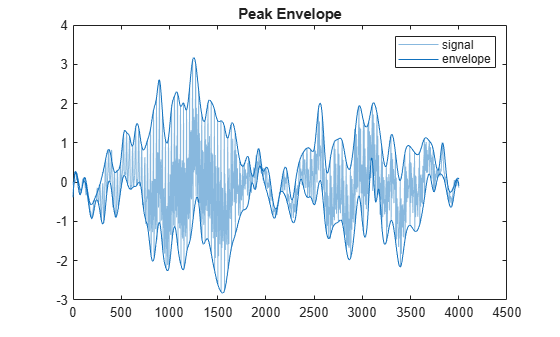Create and plot a signal that resembles the initial detection of a light pulse propagating through a dispersive medium.

```t = 0.5:-1/100:-2.49; z = airy(t*10).*exp(-t.^2); plot(z)```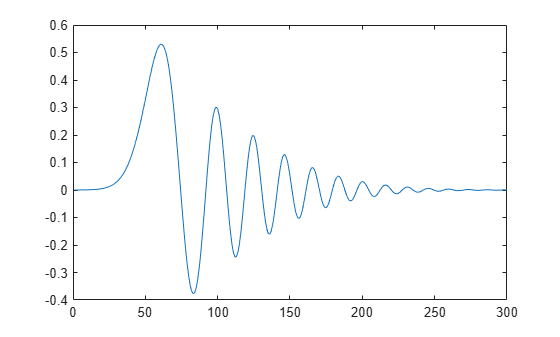Determine the envelopes of the sequence using the magnitude of its analytic signal. Plot the envelopes.

`envelope(z)`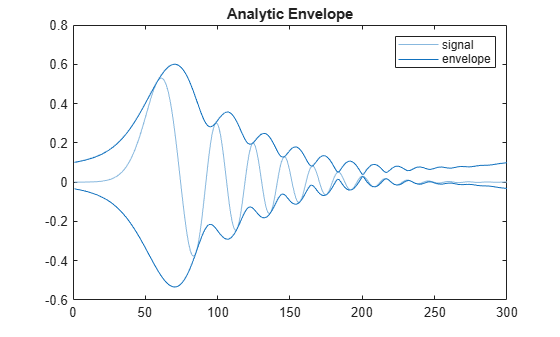Compute the analytic envelope of the signal using a 50-tap Hilbert filter.

`envelope(z,50,'analytic')`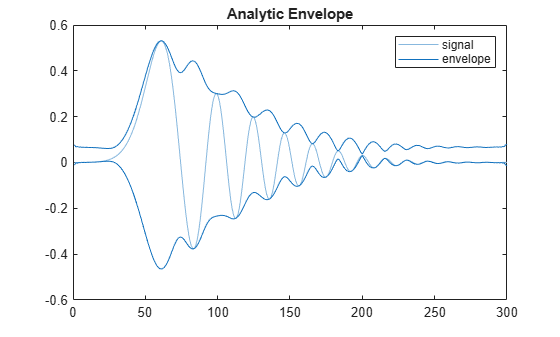Compute the RMS envelope of the signal using a 40-sample moving window. Plot the result.

`envelope(z,40,'rms')`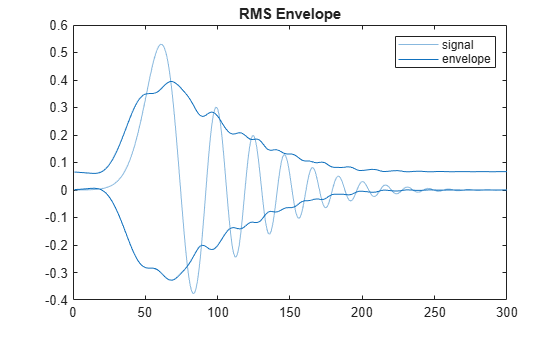Determine the peak envelopes. Use spline interpolation with not-a-knot conditions over local maxima separated by at least 10 samples.

`envelope(z,10,'peak')`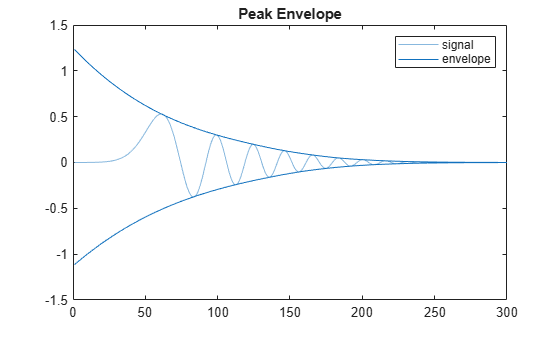## Input Arguments

collapse all

Input sequence, specified as a vector or matrix. If `x` is a vector, it is treated as a single channel. If `x` is a matrix, then `envelope` computes the envelope estimates independently for each column. All elements of `x` must be finite.

Example: `cos(pi/4*(0:159))+randn(1,160)` is a single-channel row-vector signal.

Example: `cos(pi./[4;2]*(0:159))'+randn(160,2)` is a two-channel signal.

Data Types: `single` | `double`

Hilbert filter length, specified as a positive integer scalar. The filter is created by windowing an ideal brick-wall filter with a Kaiser window of length `fl` and shape parameter β = 8.

Data Types: `single` | `double`

Window length, specified as a positive integer scalar.

Data Types: `single` | `double`

Peak separation, specified as a positive integer scalar.

Data Types: `single` | `double`

## Output Arguments

collapse all

Upper and lower signal envelopes, returned as vectors or matrices.